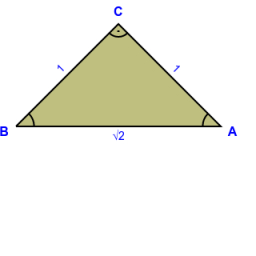# Sqrt between ints

Which two consecutive integers does the square root lie? *

√45
√23
√71
√7
√86

a45 =  6
b45 =  7
a23 =  4
b23 =  5
a71 =  8
b71 =  9
a7 =  2
b7 =  3
a86 =  9
b86 =  10

### Step-by-step explanation:Did you find an error or inaccuracy? Feel free to write us. Thank you!

Tips for related online calculators
Do you want to round the number?